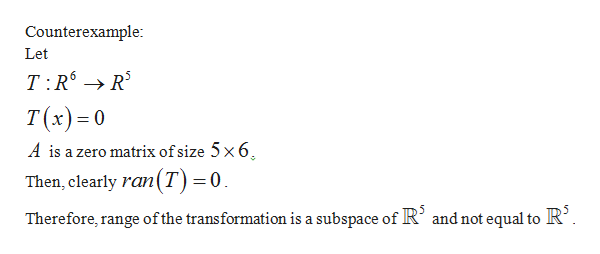# A is a 5x6 matrix, the range of transformation x->Ax must be IR^5. Is this true or False?

Question
18 views

A is a 5x6 matrix, the range of transformation x->Ax must be IR^5. Is this true or False?

check_circle

Step 1help_outlineImage TranscriptioncloseCounterexample: Let T:R → R T(x) =0 A is a zero matrix of size 5 x6. Then, clearly ran(T)=0 Therefore, range of the transformation is a subspace of R’ and not equal to R’. fullscreen

### Want to see the full answer?

See Solution

#### Want to see this answer and more?

Solutions are written by subject experts who are available 24/7. Questions are typically answered within 1 hour.*

See Solution
*Response times may vary by subject and question.
Tagged in

### Math